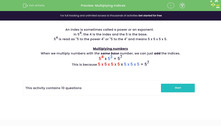# Multiply Indices

In this worksheet, students will multiply numbers expressed using indices.Key stage:  KS 3

Curriculum topic:   Number

Curriculum subtopic:   Understand Integer Powers/Real Roots

Difficulty level:#### Worksheet Overview

An index is sometimes called a power or an exponent.

In 54, the 4 is the index and the 5 is the base.

54 is read as '5 to the power 4' and means 5 x 5 x 5 x 5.

When we multiply numbers with the same base number, we can just add the indices.

54 x 53 = 57

This is because 5 x 5 x 5 x 5 x 5 x 5 x 5 = 57

Let's test this out in some questions now.

### What is EdPlace?

We're your National Curriculum aligned online education content provider helping each child succeed in English, maths and science from year 1 to GCSE. With an EdPlace account you’ll be able to track and measure progress, helping each child achieve their best. We build confidence and attainment by personalising each child’s learning at a level that suits them.

Get started